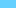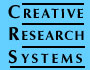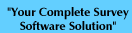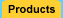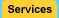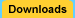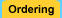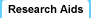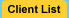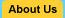Sample Size Calculator Significance Survey Design Correlation Interviewing Companies# Statistical Significance

"Significance level" is a misleading term that many researchers do not fully understand. This article may help you understand the concept of statistical significance and the meaning of the numbers produced by The Survey System.

This article is presented in two parts. The first part simplifies the concept of statistical significance as much as possible; so that non-technical readers can use the concept to help make decisions based on their data. The second part provides more technical readers with a fuller discussion of the exact meaning of statistical significance numbers.

In normal English, "significant" means important, while in Statistics "significant" means probably true (not due to chance). A research finding may be true without being important. When statisticians say a result is "highly significant" they mean it is very probably true. They do not (necessarily) mean it is highly important.

Take a look at the table below. The chi (pronounced kie like pie) squares at the bottom of the table show two rows of numbers. The top row numbers of 0.07 and 24.4 are the chi square statistics themselves. The meaning of these statistics may be ignored for the purposes of this article. The second row contains values .795 and .001. These are the significance levels and are explained following the table.

 Do you buy Brand X gasoline? Area Type of Vehicle Total City Suburb Car Truck Bus Van Base 713 361 352 247 150 44 180 Yes 428 60% 215 60% 213 61% 131 53% 74 49% 29 66% 131 73% No 285 40% 146 40% 139 40% 116 47% 76 51% 15 34% 49 27% Chi Square 0.07 .795 24.4 .001

Significance levels show you how likely a result is due to chance. The most common level, used to mean something is good enough to be believed, is .95. This means that the finding has a 95% chance of being true. However, this value is also used in a misleading way. No statistical package will show you "95%" or ".95" to indicate this level. Instead it will show you ".05," meaning that the finding has a five percent (.05) chance of not being true, which is the converse of a 95% chance of being true. To find the significance level, subtract the number shown from one. For example, a value of ".01" means that there is a 99% (1-.01=.99) chance of it being true. In this table, there is probably no difference in purchases of gasoline X by people in the city center and the suburbs, because the probability is .795 (i.e., there is only a 20.5% chance that the difference is true). In contrast the high significance level for type of vehicle (.001 or 99.9%) indicates there is almost certainly a true difference in purchases of Brand X by owners of different vehicles in the population from which the sample was drawn.

The Survey System uses significance levels with several statistics. In all cases, the p value tells you how likely something is to be not true. If a chi square test shows probability of .04, it means that there is a 96% (1-.04=.96) chance that the answers given by different groups in a banner really are different. If a t-test reports a probability of .07, it means that there is a 93% chance that the two means being compared would be truly different if you looked at the entire population.

People sometimes think that the 95% level is sacred when looking at significance levels. If a test shows a .06 probability, it means that it has a 94% chance of being true. You can't be quite as sure about it as if it had a 95% chance of being be true, but the odds still are that it is true. The 95% level comes from academic publications, where a theory usually has to have at least a 95% chance of being true to be considered worth telling people about. In the business world if something has a 90% chance of being true (probability =.1), it can't be considered proven, but it is probably better to act as if it were true rather than false.

If you do a large number of tests, falsely significant results are a problem. Remember that a 95% chance of something being true means there is a 5% chance of it being false. This means that of every 100 tests that show results significant at the 95% level, the odds are that five of them do so falsely. If you took a totally random, meaningless set of data and did 100 significance tests, the odds are that five tests would be falsely reported significant. As you can see, the more tests you do, the more of a problem these false positives are. You cannot tell which the false results are - you just know they are there.

Limiting the number of tests to a small group chosen before the data is collected is one way to reduce the problem. If this isn't practical, there are other ways of solving this problem. The best approach from a statistical point of view is to repeat the study and see if you get the same results. If something is statistically significant in two separate studies, it is probably true. In real life it is not usually practical to repeat a survey, but you can use the "split halves" technique of dividing your sample randomly into two halves and do the tests on each. If something is significant in both halves, it is probably true. The main problem with this technique is that when you halve the sample size, a difference has to be larger to be statistically significant.

The last common error is also important. Most significance tests assume you have a truly random sample. If your sample is not truly random, a significance test may overstate the accuracy of the results, because it only considers random error. The test cannot consider biases resulting from non-random error (for example a badly selected sample).

To summarize:

• In statistical terms, significant does not necessarily mean important.
• Probability values should be read in reverse (1 - p).
• Too many significance tests will turn up some falsely significant relationships.
• Check your sampling procedure to avoid bias.

## Part Two - The Exact Meaning of Statistical Significance Numbers

The preceding discussion recommends reading probability values in reverse (1 - p). Doing so will normally lead to correct decision making, but it is something of an over-simplification from the technical point of view. A more complex, technically correct discussion is presented here.

Unfortunately, statistical significance numbers do not directly tell us exactly what we want to know. They tell us how likely we would be to get differences between groups in our sample that are as large or larger than those we see, if there were no differences between the corresponding groups in the population represented by our sample. In other words, these numbers tell us how likely is our data, given the assumption that there are no differences in the population. What we want to know is how likely there are differences in the population, given our data.

Logically, if we are sufficiently unlikely to get a difference found in our sample, if there were no difference in the population, then it is likely that there is a difference in the population. We used this logic in the first part of this article when we said that you can interpret significance numbers by considering 1-p as the probability that there is a difference in the population (where p is the significance number produced by the program). For example, if the significance level is .05 then you could consider the likelihood that there is a difference in the population to be 95% (1-.05).

While this logic passes the common sense test, the mathematics behind statistical significance do not actually guarantee that 1-p gives the exact probability that there is a difference is the population. Even so, many researchers treat 1-p as that probability anyway for two reasons. One is that no one has devised a better general-purpose measure. The other is that using this calculation will usually lead one to a useful interpretation of statistical significance numbers.

In some non-survey fields of research, the possibility that 1-p is not the exact probability that there is a difference in the population may be more important. In these fields, the use of statistical significance numbers may be controversial.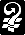Copyright © 2000  Creative Research Systems  (707) 765-1001  info@surveysystem.com